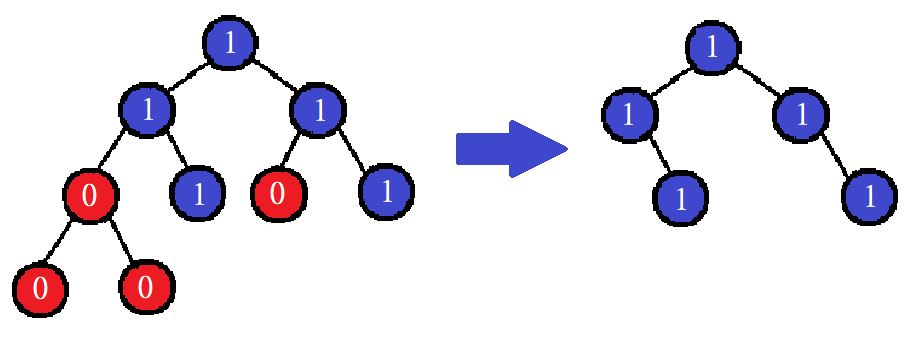# Binary Tree Pruning

Posted: 12 Sep, 2020
Difficulty: Moderate

## PROBLEM STATEMENT

#### Note :

``````A subtree of a node X is X, plus every node that is a descendant of X.
``````

#### For Example :

``````Look at the below example to see a Binary Tree pruning.
Input: [1, 1, 1, 0, 1, 0, 1, 0, 0, -1, -1, -1, -1, -1, -1, -1, -1, -1, -1]
````````````Output: [1, 1, 1, -1, 1, -1, 1, -1, -1, -1, -1]

For example, the input for the tree depicted in the below image would be :
````````````1
2 3
4 -1 5 6
-1 7 -1 -1 -1 -1
-1 -1
``````

#### Explanation :

``````Level 1 :
The root node of the tree is 1

Level 2 :
Left child of 1 = 2
Right child of 1 = 3

Level 3 :
Left child of 2 = 4
Right child of 2 = null (-1)
Left child of 3 = 5
Right child of 3 = 6

Level 4 :
Left child of 4 = null (-1)
Right child of 4 = 7
Left child of 5 = null (-1)
Right child of 5 = null (-1)
Left child of 6 = null (-1)
Right child of 6 = null (-1)

Level 5 :
Left child of 7 = null (-1)
Right child of 7 = null (-1)

The first not-null node (of the previous level) is treated as the parent of the first two nodes of the current level. The second not-null node (of the previous level) is treated as the parent node for the next two nodes of the current level and so on.
The input ends when all nodes at the last level are null (-1).
``````

#### Note :

``````The above format was just to provide clarity on how the input is formed for a given tree.

The sequence will be put together in a single line separated by a single space. Hence, for the above-depicted tree, the input will be given as:
1 2 3 4 -1 5 6 -1 7 -1 -1 -1 -1 -1 -1
``````

#### Input Format :

``````The first line contains an integer 'T' which denotes the number of test cases or queries to be run. Then the test cases follow.

The only line of each test case contains elements in the level order form. The line consists of values of nodes separated by a single space. In case a node is null, we take -1 on its place.
``````

#### Output Format :

``````Print the level order traversal of the pruned binary tree.

Print output of each test case in a separate line.
``````

#### Note :

``````You are not required to print the expected output, it has already been taken care of. Just implement the function.
``````

#### Constraints :

``````1 <= T <= 10^2
0 <= N <= 10^3
0 <= data <= 1

Where 'N' is the number of nodes in the tree and 'data' is the value of nodes.

Time Limit: 1 sec
``````Approach 1

If we have to process a subtree from the tree, it is better to process the child subtrees first i.e. PostOrder Traversal. This strategy will help us in two ways-

• We can keep track if a 1 is present in the subtree or not.
• If a 1 is not present in a subtree, we can delete it directly (by assign ‘NULL’ to that subtree).

Here, is the complete recursive algorithm

• Create a hasOne function, which tells if a subtree has a 1 or not.
• Check if the left and right subtrees have a 1 or not.
• If the left subtree doesn’t have a 1, set the left child as NULL.
• If the right subtree doesn’t have a 1, set the right child as NULL.
• If either of the left or right subtrees or the current node have 1, Return true. Else, return false.